# Introduction to Quadratics in Trigonometry: Tutoring Solution Chapter Exam

Exam Instructions:

Choose your answers to the questions and click 'Next' to see the next set of questions. You can skip questions if you would like and come back to them later with the yellow "Go To First Skipped Question" button. When you have completed the practice exam, a green submit button will appear. Click it to see your results. Good luck!

### Page 1

#### Question 2 2. Use the quadratic formula to find the solutions to the equation below.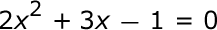#### Question 4 4. Which of the following are the solutions to the equation below?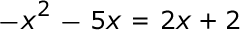#### Question 5 5. Which statement is true about the inequality below?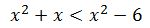### Page 2

#### Question 7 7. Rewrite the quadratic below in standard form: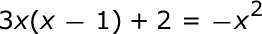### Page 3

#### Question 11 11. Find the roots of the equation.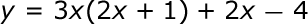#### Question 12 12. Find the roots of the equation below.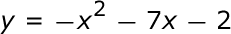#### Question 13 13. What is the b-value of the quadratic equation below?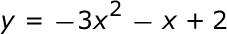### Page 4

#### Question 16 16. What are the zeros in the following quadratic equation?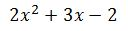#### Question 20 20. Solve the equation.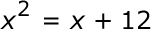### Page 5

#### Question 21 21. Solve the following inequality.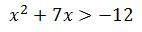#### Question 22 22. Solve this inequality.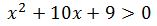#### Introduction to Quadratics in Trigonometry: Tutoring Solution Chapter Exam Instructions

Choose your answers to the questions and click 'Next' to see the next set of questions. You can skip questions if you would like and come back to them later with the yellow "Go To First Skipped Question" button. When you have completed the practice exam, a green submit button will appear. Click it to see your results. Good luck!

Support